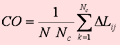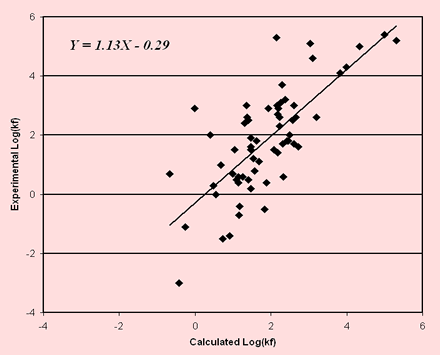K-Fold Predictor of the Protein Folding Mechanism and Rate
 Last Update 04/09/13

K-Fold: A Tool for the Prediction
of the
Protein Folding Kinetic Order and Rate

Introduction

K-Fold is a tool for the automatic prediction of protein folding characteristics. The tool is based on a support vector machine-based and it was trained on the data set of 63 proteins, whose folding mechanism has been experimentally detected and described in previous publications. K-Fold can be use both as classifier, predicting the kinetic order of folding process and as direct predictor of the logarithm of the folding rate. The method correctly classifies 81% of the folding mechanisms over the set of the 63 experimental data and predicts whether the protein folds according to a two-state or a multi-state kinetics. Secondly we focus on the prediction of the logarithm of the folding rate. This value can be obtained as a linear regression task again with a SVM-based method, implemented in the same web tool. To the best of our knowledge, the tool discriminates for the first time starting from the protein sequence and contact order, whether a protein is characterized by a two state or a multiple state kinetics, during the folding process, and concomitantly estimates also the value of the constant rate of the process.
When used to predict the logarithm of the folding rate, K-Fold scores with correlation value to the experimental data of 0.74. The web interface is interactive requires either the upload of a pdb file or any of the codes of the Protein Data Bank and it is user friendly. Furthermore it also accepts protein fragments of any required length. The job running time depends on the protein length and routinely for the longest proteins does not exceed 1 minute.

K-Fold Description

K-Fold was trained to accomplish two different tasks:

1) prediction of the kinetic order of the folding process (a classification task);
2) prediction of the log(kf) value of the folding process (a function approximation task);

For each task, K-Fold is based on support vector machines (SVM). We tested several kernels and we found that the most convenient for the problems at hand is the one based on Linear Functions (Linear kernel function K(xi,xj) = xi K xj ). The results here described are therefore relative only to the linear kernel. For the classification task and for assigning the log(kf) values we basically adopt a similar input code by identifying two labels: one represents the protein that folds without intermediate states (two-state kinetic, label is TS), the other with one or more intermediates states (multi-state kinetic, label is MS). The input vector consists of 2 values. The first input value accounts for the natural logarithm of the chain length (number of residues) and the second for the protein relative contact order (CO). CO is calculated starting from the protein structure with the following equation:(1)

where N is the number of amino acid residues, Nc is total number of contacts and DLij = |i-j| is the sequence separation between contacting residues i and j. After a search in the parameter space, our procedure considers to be in contact all the residues that have at least one couple of heavy atoms with a distance below 0.9 nm. We found that the classification between TS and MS proteins improves when considering only those contacts between those residue pairs with a sequence separation larger than 6. This suggests that long range interactions may be relevant in discriminating between the two different folding processes. On the other hand, it is known that the protein folding constant rate is a more general property of the folding process, depending on local as well global interactions. Interestingly, and in line with this view, in the regression task for the evaluation of the logarithm of the folding rate optimal scoring is found when considering all the contacts without sequence separation cut-off. According to this, the inputs of the two SVMs, trained to address the classification and the regression tasks, respectively, consist of two different values of CO, calculated starting from the same protein structure using either w=6 or w=0, depending on the task at hand (for more details see http://arxiv.org/abs/q-bio.BM/0602013 )

Results

The scoring efficiency of the method is evaluated by computing the scoring indexes listed below:

 Q2 P(TS) Q(TS) P(MS) Q(MS) C K-Fold 0.81 0.83 0.87 0.78 0.72 0.60

The overall accuracy Q2 is:

Q2=p/N

where p is the total number of correctly predicted residues and N is the total number of residues.
The correlation coefficient C is defined as:

C(s)=[ p(s)n(s)-u(s)o(s) )] / D

where D is the normalization factor

D =[(p(s)+u(s))(p(s)+o(s))(n(s)+u(s))(n(s)+o(s))]1/2

for each class s (TS and MS, for two-state and multi-state kinetic order respectively); p(s) and n(s) are the total number of correct predictions and correctly rejected assignments, respectively; u(s) and o(s) are the numbers of under and over predictions.

The coverage for each discriminated structure s is evaluated as:

Q(s)=p(s)/[ p(s)+u(s)]

where p(s) and u(s) are as defined above. The probability of correct predictions P(s) (or accuracy for s) is computed as:

P(s)=p(s) / [p(s) + o(s)]

where p(s) and o(s) are previously defined (ranging from 1 to 0).

K-Fold predicts also the value of the decimal logarithm of the folding rate. In this particular task it reaches a correlation with the experimental data of 0.74 (see figure below) when structural information is considered (associated Standard Error is 1.2).Required Inputs

K-Fold is optimized to predict the protein folding kinetics and rate starting from the PDB protein structure or uploading a pdb file. The following inputs:

• PDB code: the PDB protein code  or PDB File: uploadable structural file
• Chain: if the input is a PDB file containing more than one chain, the chain label is also necessary; otherwise the default value is "_";
• Residues: the first and the last residues separated by "-" considered;
• Predict: the user can predict the kinetic order, the logarithm of the folding rate or both

For either prediction the option is to predict the kinetic order of the folding process (two-state TS, multi-state MS) or the logarithm of the folding rate (log[kf]) . The results can be sent to your e-mail address, if you ask for it, or obtained interactively if you do not past your e-mail in the proper box.

Outputs

The common output consists of a table listing the first and the last residue of the considered PDB structure, the length of the provided protein or fragment, the Contact Order calculated using two different sequence separation (0 and 6) (as explained above) . If the prediction of the kinetic order is required the last part of the output will be TS for two state or MS for multi-state protein kinetic type and the associated reliability index. When the Folding Rate option is selected the output will contain the logarithm of the folding rate.
A brief description of the output can be summarized as follows

Residues: First-Last residues
Length: Chain Length
CO[W]: Contact Order calculated using a sequence separation W
RI: Reliability Index that is computed only when the kinetic order of the folding process is predicted and is evaluated from the output of the support vector machine O as RI=20*abs(O-0.5).
States: Kinetic Mechanisms of the Protein Folding (TS: Two-State or MS: Multiple-State)
log[Kf]: Logarithm of the Folding Rate

WARNING:
Possible errors may occur when the PDB files contain broken chains or the numbering for the selected residue is different from than expected by the user.

 Berman HM, Westbrook J, Feng Z, Gilliland G, Bhat TN, Weissig H, Shindyalov IN, Bourne PE (2000). The Protein Data Bank. Nucleic Acids Res. 28, 235-242.